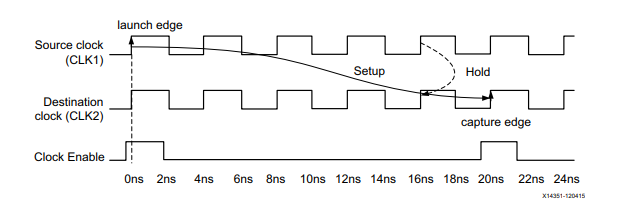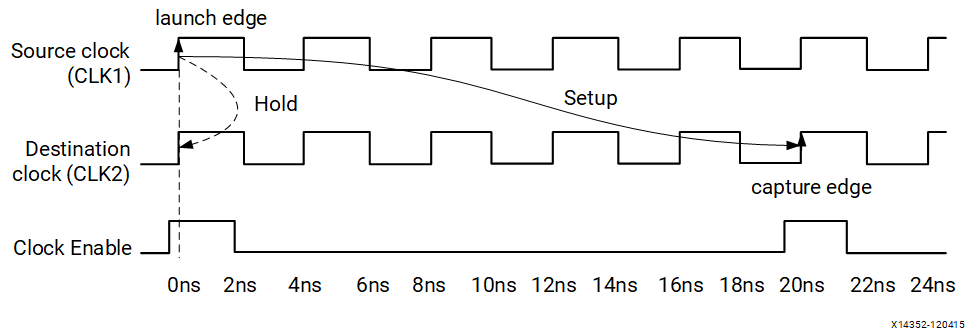# Example Two: Setup=5 / Hold=4 - 2022.1 English

## Vivado Design Suite User Guide: Using Constraints (UG903)

Document ID
UG903
Release Date
2022-06-01
Version
2022.1 English

This example assumes that the following are defined:

• A setup multiplier of five (5)
• A hold multiplier of four (4) (that is, 5-1)

This corresponds to a transfer between two sequential cells when a new data is launched and captured every five (5) cycles.

``````set_multicycle_path 5 -setup -from [get_pins data0_reg/C] -to [get_pins data1_reg/D]
set_multicycle_path 4 -hold -from [get_pins data0_reg/C] -to [get_pins data1_reg/D]``````

By default, the setup multiplier is applied against the destination clock, which in this case results in moving the capture edge forward to the fifth cycle instead of the first cycle.

Accordingly, by default, the hold check follows the setup check.

On specifying the second command, the hold multiplier is applied against the source clock, which in this case results in moving the launch edge forward to the fourth cycle.

Figure 1. Setup=5, Hold=4Because both source and destination clocks have the same waveforms, and are phase-aligned, Figure 1 is equivalent to Figure 2.

Figure 2. Setup=5, Hold=4Important: In general, within a clock domain or between two clocks with the same waveform, when a setup multiplier of N is defined, define a hold multiplier of N-1 (most common case) as shown below.
``````set_multicycle_path N -setup -from [get_pins data0_reg/C] -to [get_pins data1_reg/D]
set_multicycle_path N-1 -hold -from [get_pins data0_reg/C] -to [get_pins data1_reg/D]``````